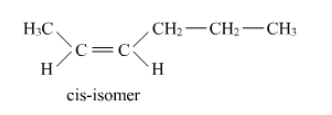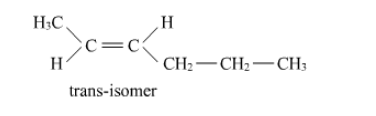# Draw the cis and trans structures of hex-2-ene.

Question:

Draw the cis and trans structures of hex-2-ene. Which isomer will have higher b.p. and why?

Solution:

Hex-2-ene is represented as:

$\mathrm{CH}_{3}-\mathrm{CH}=\mathrm{CH}-\mathrm{CH}_{2}-\mathrm{CH}_{2}-\mathrm{CH}_{3}$

Geometrical isomers of hex-2-ene are:The dipole moment of cis-compound is a sum of the dipole moments of $\mathrm{C}-\mathrm{CH}_{3}$ and $\mathrm{C}-\mathrm{CH}_{2} \mathrm{CH}_{2} \mathrm{CH}_{3}$ bonds acting in the same direction.

The dipole moment of trans-compound is the resultant of the dipole moments of $\mathrm{C}-\mathrm{CH}_{3}$ and $\mathrm{C}-\mathrm{CH}_{2} \mathrm{CH}_{2} \mathrm{CH}_{3}$ bonds acting in opposite directions.

Hence, cis-isomer is more polar than trans-isomer. The higher the polarity, the greater is the intermolecular dipole-dipole interaction and the higher will be the boiling point. Hence, cis-isomer will have a higher boiling point than trans-isomer.